CFA财务报表分析练习题＂Financial Report＂：Rev-aluation model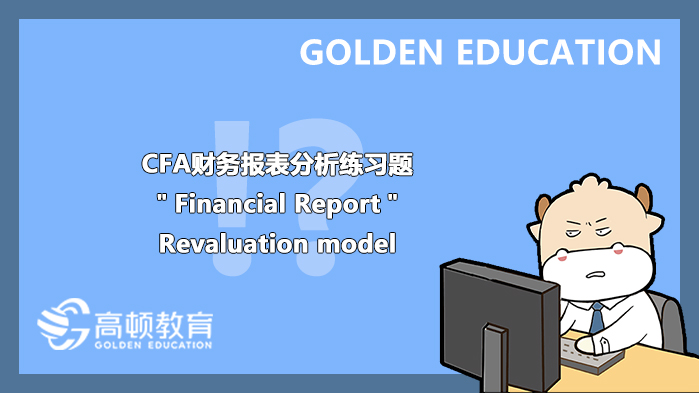### Questions 1:

A company that reports in accordance with IFRS does not use the cost model to value its investment properties and property,plant,and equipment.Information related to an investment property and a plant is as follows:The impact on its net income for the year will most likely be a gain(in thousands)of:
A、€100.
B、€300.
C、€200.

#### 【Answer to question 1】A

【analysis】
A is correct.The fair value model would be used for the investment property,and the€100 thousand gain should be recognized on the company’s income statement.The r*uation model would be used for the plant,and the€200 thousand gain should be recognized in the r*uation surplus account on the balance sheet with no impact on net income.Therefore,only the€100 thousand will affect net income.
B is incorrect.The r*uation model would be used for the plant,and the gain should be recognized in the r*uation surplus account on the balance sheet.This is a new purchase and therefore no gains need to be recognized on the income statement to reverse previously recognized losses.Therefore,a maximum of\$100,000 would be recognized on the income statement.
C is incorrect.The r*uation model would be used for the plant,and the gain should be recognized in the r*uation surplus account on the balance sheet.This is a new purchase and therefore no gains need to be recognized on the income statement to reverse previously recognized losses.

### Questions 2:

The following is selected balance sheet data for a company along with information about its financial and operating lease obligations.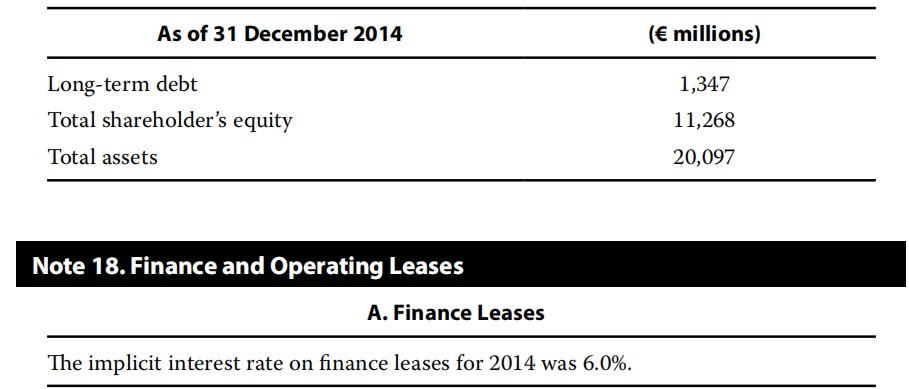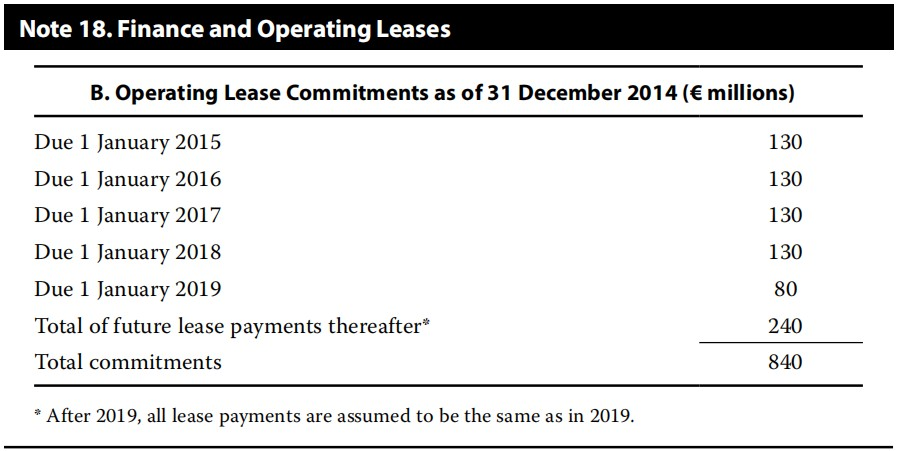If the company were to capitalize its long-term leases,its adjusted long-term debt-to-assets ratio as of the end of December 2014 would be closest to:
A、9.9%.
B、10.2%.
C、10.4%.

#### 【Answer to question 2】A

【analysis】
A is correct.If the leases were capitalized,both total assets and liabilities would increase by the present value of the lease payments.The lease commitments after 2019 are assumed to be the same as in 2019,so there are estimated to be 240/80=3 additional payments.The present value of the operating lease payments can be calculated as the sum of the present values of two annuities-in-advance(PVAADV):a four-year annuity starting immediately(beginning of 2015)and another four-year annuity starting in four years(2019)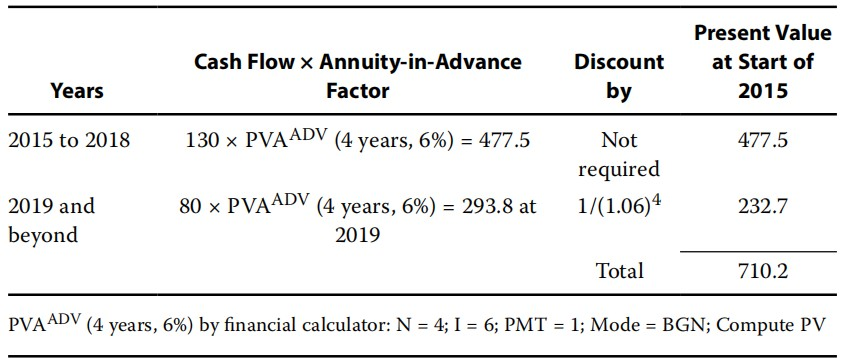Adjusted long-term debt/asset ratio calculation:
Adjusted long-term debt:1,347+710=2,057
Adjusted total assets:20,097+710=20,807
Adjusted long-term debt/asset ratio:2,057/20,807=9.9%
Alternatively,the individual cash flows can be separately discounted.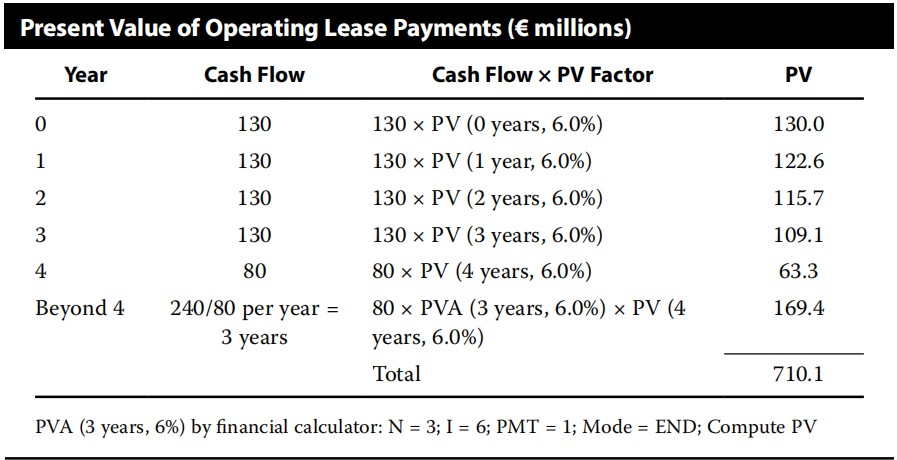B is incorrect.Adding PV(Operating lease)only to debt:2,057/20,097=10.2%.
C is incorrect.The undiscounted total commitments are added to both assets and long-term debt:Adjusted debt ratio=(1,347+840)/(20,097+840)=10.4%